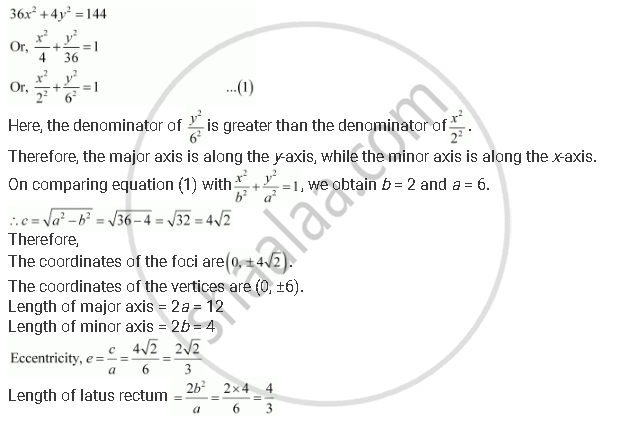# Find the Coordinates of the Foci, the Vertices, the Length of Major Axis, the Minor Axis, the Eccentricity and the Length of the Latus Rectum of the Ellipse 36x2 + 4y2 = 144 - Mathematics

Find the coordinates of the foci, the vertices, the length of major axis, the minor axis, the eccentricity and the length of the latus rectum of the ellipse 36x2 + 4y2 = 144

#### Solution

The given equation is 36x2 + 4y2 = 144.

It can be written asConcept: Ellipse - Relationship Between Semi-major Axis, Semi-minor Axis and the Distance of the Focus from the Centre of the Ellipse
Is there an error in this question or solution?
Chapter 11: Conic Sections - Exercise 11.3 [Page 255]

#### APPEARS IN

NCERT Class 11 Mathematics
Chapter 11 Conic Sections
Exercise 11.3 | Q 7 | Page 255
Share